## Metaphysics on geometric distribution in probability theory

I realized geometric distribution is not exactly about the time needed to get the first success in a given number of trials. This is a very odd feeling. It is probably a feeling applied mathematicians get sometimes, when they feel they are doing the best they can, and yet the theory is not perfect.

This may be a naive post, I warn you, but I was really stunned when I realized this.

### Geometric distribution is not about the first success

Let’s jump to the point. We know (or at least, I was taught) that geometric distribution is used to calculate the probability that the first success in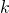trials (all independent and of probability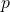) will happen precisely at the-th trial.

Remember that a geometric distribution is a random variable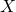such that its distribution is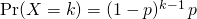How can we relate the above distribution with the fact that it matches the first success? Well, we need to have one success, which explains theat the bottom. Moreover, we want to have just one success, so all other trials must be unsuccessful, which explains the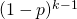.

But hey, where would first ever be written? Continue reading “Metaphysics on geometric distribution in probability theory”

## Random variables: what are they and why are they needed?

This article aims at providing some intuition for what random variables are and why random variables are useful and needed in probability theory.

## Intuition for random variables

Informally speaking, random variables encode questions about the world in a numerical way.

How many heads can I get if I flip a coin 3 times?

How many people will vote the Democrats at the US presidential elections?

I want to make pizza. What is the possible overall cost of the ingredients, considering all combinations of different brands of them?

These are all examples of random variables. What a random variable does, in plain words, is to take a set of possible world configurations and group them to a number. What I mean when I say world configurations will be clearer soon, when talking about the sample space(which, appropriately, is also called universe).